# Math2.org Math Tables: Special Functions(Math)Some of these functions I have seen defined under both intervals (0 to x) and (x to inf). In that case, both variant definitions are listed.
gamma = Euler's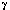constant = 0.5772156649...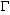(x) =tx-1 e-t dt (Gamma function)

B(x,y) =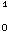tx-1 (1-t)y-1dt (Beta function)

Ei(x) =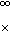e-t/t dt (exponential integral) or it's variant, NONEQUIVALENT form:

Ei(x) =+ ln(x) +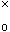(e^t - 1)/t dt = gamma + ln(x) +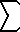(n=1..inf)x^n/(n*n!)
li(x) =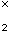1/ln(t) dt (logarithmic integral)
Si(x) =sin(t)/t dt (sine integral) or it's variant, NONEQUIVALENT form:
Si(x) =sin(t)/t dt = PI/2 -sin(t)/t dt

Ci(x) =cos(t)/t dt (cosine integral) or it's variant, NONEQUIVALENT form:
Ci(x) = -cos(t)/t dt = gamma + ln(x) +(cos(t) - 1) / t dt (cosine integral)

Chi(x) = gamma + ln(x) +(cosh(t)-1)/t dt (hyperbolic cosine integral)
Shi(x) =sinh(t)/t dt (hyperbolic sine integral)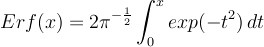(error function)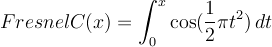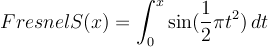dilog(x) =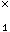ln(t) (1-t)-1 dt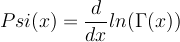Psi(n,x) = nth derivative of Psi(x)

W(x) = inverse of x ex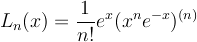(laguerre polynomial degree n. (n) meaning nth derivative)Dirichlet's beta functionTheorems with hyperlinks have proofs, related theorems, discussions, and/or other info.